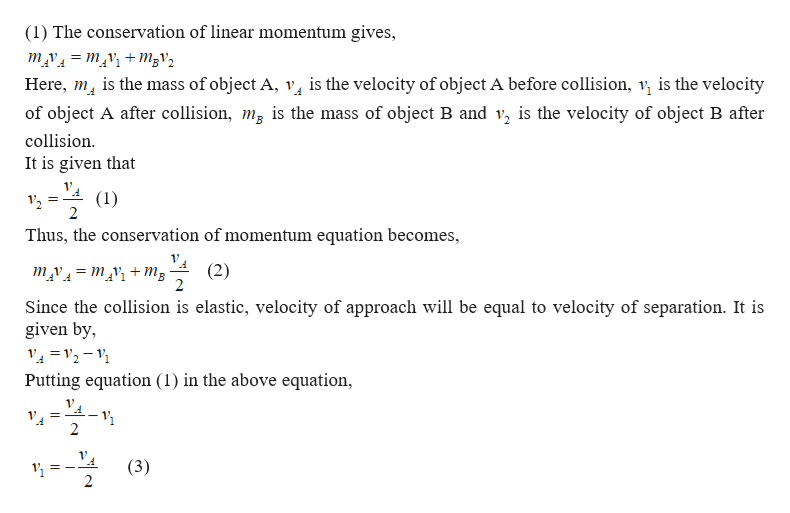# Object B is at rest when object A collides with it. The collision is one-dimensional and elastic. After the collision object B has half the velocity that object A had before the collision.1.) Which object has the greater mass?2.) By how much is the mass of object B greater than that of object A?3.) If the velocity of object A before the collision was 6.0 m/s to the right, what is its velocity after the collision?

Question
163 views

Object B is at rest when object A collides with it. The collision is one-dimensional and elastic. After the collision object B has half the velocity that object A had before the collision.

1.) Which object has the greater mass?

2.) By how much is the mass of object B greater than that of object A?

3.) If the velocity of object A before the collision was 6.0 m/s to the right, what is its velocity after the collision?

check_circle

star
star
star
star
star
1 Rating
Step 1help_outlineImage Transcriptionclose(1) The conservation of linear momentum gives, mv mv +m3v2 Here, m is the mass of object A, v is the velocity of object A before collision, v is the velocity of object A after collision, mg is the mass of object B and v, is the velocity of object B after collision It is given that (1) 12 2 Thus, the conservation of momentum equation becomes, (2) m v=m+mB Since the collision is elastic, velocity of approach will be equal to velocity of separation. It is given by, 2- Putting equation (1) in the above equation 2 1 (3) fullscreen

### Want to see the full answer?

See Solution

#### Want to see this answer and more?

Solutions are written by subject experts who are available 24/7. Questions are typically answered within 1 hour.*

See Solution
*Response times may vary by subject and question.
Tagged in
SciencePhysics

### Collisions